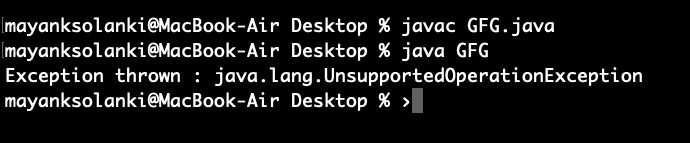# Arrays asList() method in Java with Examples

• Difficulty Level : Easy
• Last Updated : 24 Nov, 2021

The asList() method of java.util.Arrays class is used to return a fixed-size list backed by the specified array. This method acts as a bridge between array-based and collection-based APIs, in combination with Collection.toArray(). The returned list is serializable and implements RandomAccess.

Tip: This runs in O(1) time.

Syntax:

`public static List asList(T... a)`

Parameters: This method takes the array a which is required to be converted into a List.  Here … is known as varargs which is an array of parameters and works similar to an object array parameter.

Special Note: The type of array must be a Wrapper Class(Integer,Float, etc) in case of primitive data types(int, float,etc) , i.e you can’t pass int a[] but you can pass Integer a[]. If you pass int a[], this function will return a  List <int a[]> and not List <Integer> , as “autoboxing” doesn’t happen in this case and int a[] is itself identified as an object and a List of int array is returned, instead of list of integers , which will give error in various Collection functions .

Return Value: This method returns a list view of the specified array.

Example 1:

## Java

 `// Java program to Demonstrate asList() method``// of Arrays class for a string value` `// Importing utility classes``import` `java.util.*;` `// Main class``public` `class` `GFG {` `    ``// Main driver method``    ``public` `static` `void` `main(String[] argv) ``throws` `Exception``    ``{` `        ``// Try block to check for exceptions``        ``try` `{` `            ``// Creating Arrays of String type``            ``String a[]``                ``= ``new` `String[] { ``"A"``, ``"B"``, ``"C"``, ``"D"` `};` `            ``// Getting the list view of Array``            ``List list = Arrays.asList(a);` `            ``// Printing all the elements in list object``            ``System.out.println(``"The list is: "` `+ list);``        ``}` `        ``// Catch block to handle exceptions``        ``catch` `(NullPointerException e) {` `            ``// Print statement``            ``System.out.println(``"Exception thrown : "` `+ e);``        ``}``    ``}``}`

Output

`The list is: [A, B, C, D]`

Example 2:

## Java

 `// Java program to Demonstrate asList() method``// of Arrays class For an integer value` `// Importing utility classes``import` `java.util.*;` `// Main class``public` `class` `GFG {` `    ``// Main driver method``    ``public` `static` `void` `main(String[] argv) ``throws` `Exception``    ``{``        ``// Try block to check for exceptions``        ``try` `{` `            ``// Creating Arrays of Integer type``            ``Integer a[] = ``new` `Integer[] { ``10``, ``20``, ``30``, ``40` `};` `            ``// Getting the list view of Array``            ``List list = Arrays.asList(a);` `            ``// Printing all the elements inside list object``            ``System.out.println(``"The list is: "` `+ list);``        ``}` `        ``// Catch block to handle exceptions``        ``catch` `(NullPointerException e) {` `            ``// Print statements``            ``System.out.println(``"Exception thrown : "` `+ e);``        ``}``    ``}``}`

Output

`The list is: [10, 20, 30, 40]`

Example 3:

## Java

 `// Java Program to demonstrate asList() method``// Which returns fixed size list and``// throws UnsupportedOperationException``// if any element is added using add() method` `// Importing required classes``import` `java.util.*;` `// Main class``public` `class` `GFG {` `    ``// Main driver method``    ``public` `static` `void` `main(String[] argv) ``throws` `Exception``    ``{``        ``// Try block to check for exceptions``        ``try` `{` `            ``// Creating Arrays of Integer type``            ``Integer a[] = ``new` `Integer[] { ``10``, ``20``, ``30``, ``40` `};` `            ``// Getting the list view of Array``            ``List list = Arrays.asList(a);` `            ``// Adding another int to the list``            ``// As Arrays.asList() returns fixed size``            ``// list, we'll get``            ``// java.lang.UnsupportedOperationException``            ``list.add(``50``);` `            ``// Printing all the elements of list``            ``System.out.println(``"The list is: "` `+ list);``        ``}` `        ``// Catch block to handle exceptions``        ``catch` `(UnsupportedOperationException e) {` `            ``// Display message when exception occurs``            ``System.out.println(``"Exception thrown : "` `+ e);``        ``}``    ``}``}`

Output:My Personal Notes arrow_drop_up